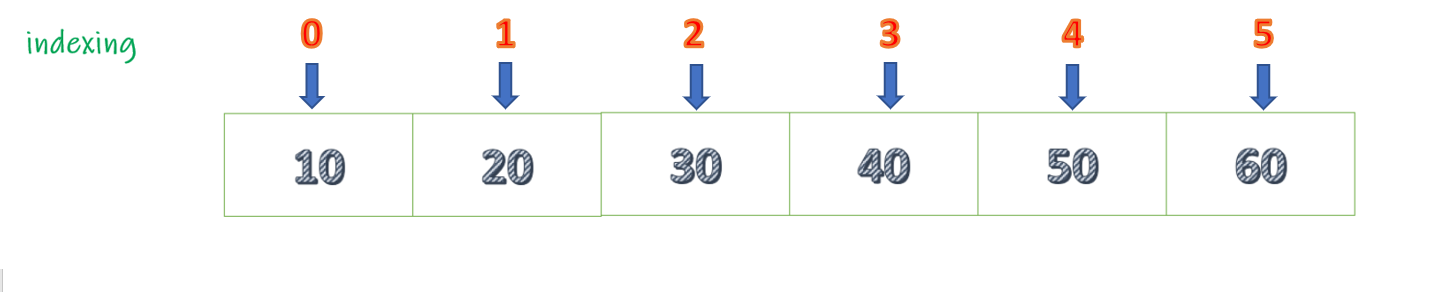# Java Program to Return the Elements at Odd Positions in a List

• Last Updated : 17 Dec, 2020

Given a List, the task is to return the elements at Odd positions in a list. Let’s consider the following list.Clearly, we can see that elements 20, 40, 60 are at Odd Positions as the index of the list is zero-based. Now we should return these elements.

Approach 1:

• Initialize a temporary value with zero.
• Now traverse through the list.
• At each iteration check the temporary value if value equals to odd then return that element otherwise just continue.
• After each iteration increment the temporary value by 1.
• However, this can be done without using temporary value. Since the data in the list is stored using fixed index therefore we can directly check if the index is odd or even and return the element accordingly

Example:

## Java

 `// Java Program to Return the Elements ` `// at Odd Positions in a List ` `import` `java.io.*; ` `import` `java.util.*; ` ` `  `class` `GFG { ` `   `  `    ``public` `static` `void` `main(String[] args) ` `    ``{ ` `        ``// Creating our list from above illustration ` `        ``List my_list = ``new` `ArrayList(); ` `        ``my_list.add(``10``); ` `        ``my_list.add(``20``); ` `        ``my_list.add(``30``); ` `        ``my_list.add(``40``); ` `        ``my_list.add(``50``); ` `        ``my_list.add(``60``); ` ` `  `        ``// creating a temp_value for checking index ` `        ``int` `temp_val = ``0``; ` ` `  `        ``// using a for-each loop to ` `          ``// iterate through the list ` `        ``System.out.print(``"Elements at odd position are : "``); ` `        ``for` `(Integer numbers : my_list) { ` `            ``if` `(temp_val % ``2` `!= ``0``) { ` `                ``System.out.print(numbers + ``" "``); ` `            ``} ` `            ``temp_val += ``1``; ` `        ``} ` `    ``} ` `}`

Output

`Elements at odd position are : 20 40 60`

Approach 2:

• Traverse the list starting from position 1.
• Now increment the position by 2 after each iteration. By doing this we always end up in an odd position.
• Iteration 1: 1+2=3
• Iteration 2: 2+3=5
• Iteration 3: 5+2=7
• And so on.
• Return the value of the element during each iteration.

Example:

## Java

 `// Java Program to Return the Elements ` `// at Odd Positions in a List ` ` `  `import` `java.io.*; ` `import` `java.util.*; ` ` `  `class` `GFG { ` `   `  `    ``public` `static` `void` `main(String[] args) ` `    ``{ ` `        ``// creating list from above illustration ` `        ``List my_list = ``new` `ArrayList<>(); ` `        ``my_list.add(``10``); ` `        ``my_list.add(``20``); ` `        ``my_list.add(``30``); ` `        ``my_list.add(``40``); ` `        ``my_list.add(``50``); ` `        ``my_list.add(``60``); ` ` `  `        ``// iterating list from position one and incrementing ` `        ``// the index value by 2 ` `        ``System.out.print( ` `            ``"Elements at odd positions are : "``); ` `       `  `        ``for` `(``int` `i = ``1``; i < ``6``; i = i + ``2``) { ` `            ``System.out.print(my_list.get(i) + ``" "``); ` `        ``} ` `    ``} ` `}`

Output

`Elements at odd positions are : 20 40 60`

My Personal Notes arrow_drop_up
Recommended Articles
Page :